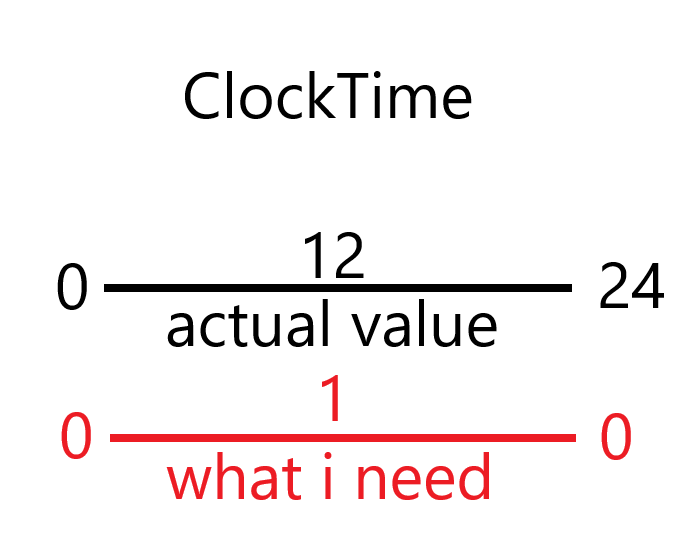# How do I get a value related to clockTime?

So I need a value that peaks at the middle of the lightings clockTime (12).The actual value of clock time goes from 0, 12, 24.
What I need is a value that goes from 0, 1 ,0.

In either a while true do loop, or a function that checks if timeofday changes:

``````value = actual/24
if value is >.5 then
need = (1-value) * 2
else
need = value * 2
end
print(need)
``````
1 Like

So im guessing that actual is the time of day, and value is the actual/24, but what is need?